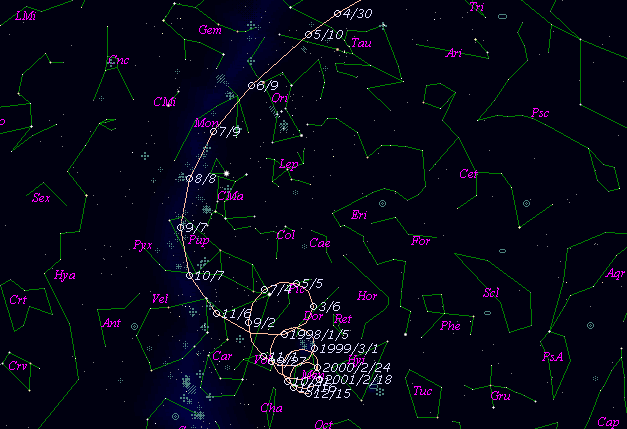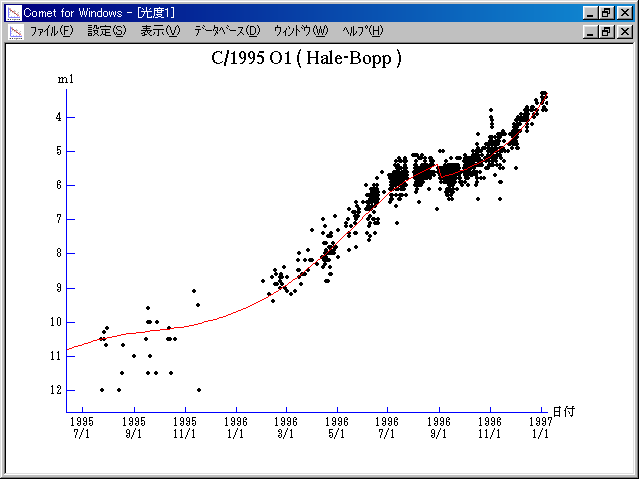# C/1995 O1 ( Hale-Bopp )###Profile

 Discovery Date July 23, 1995 Magnitude 10.5 mag, 10.8 mag Discoverer Alan Hale (Cloudcroft, NM), Thomas Bopp (Stanfield, AZ)

###Pictures###Orbital Elements

```Epoch 1997 Mar. 13.0 TT = JDT 2450520.5
T 1997 Apr. 1.13727 TT                                  MPCW
q   0.9141407            (2000.0)            P               Q
z  +0.0053998      Peri.  130.58879     -0.13312075     -0.17030406
Node   282.47071     +0.28229358     +0.93780525
e   0.9950638      Incl.   89.43006     +0.95004695     -0.30251916
From 3093 observations 1995 July 24-2013 Aug. 13, mean residual 0".8.
Nongravitational parameters A1 = +1.36, A2 = +0.0894.
```

###Finding Charts###Magnitudes Graph

```        m1 = -10   + 5 log d + 20   log r  [    ,-520]  (             - 1995 Oct. 29)
m1 =  -2.0 + 5 log d + 10.0 log r  [-520,-215]  (1995 Oct. 29 - 1996 Aug. 29)
m1 =  -0.3 + 5 log d +  7.5 log r  [-215, -35]  (1996 Aug. 29 - 1997 Feb. 25)
m1 =  -0.7 + 5 log d + 16.9 log r  [ -35,   0]  (1997 Feb. 25 - 1997 Apr.  1)
m1 =  -1.0 + 5 log d +  7.9 log r  [   0, 200]  (1997 Apr.  1 - 1997 Oct. 18)
m1 =  -1.3 + 5 log d + 10.0 log r  [ 200, 450]  (1997 Oct. 18 - 1998 June 25)
m1 =  -2.0 + 5 log d + 10.0 log r  [ 450,2250]  (1998 June 25 - 2003 May  30)
m1 = -14.5 + 5 log d + 20   log r  [2250,    ]  (2003 May  30 -             )
```##### The orbital elements are published on M.P.E.C. 2017-T14. The charts are made with StellaNavigator Ver.2.0 for Windows (AstroArts / ASCII). The magnitudes graphs are made with Comet for Windows.